Skip Counting (2s, 5s, 10s & 100s) | Grades Kindergarten, 1, & 2
1%
It was processed successfully!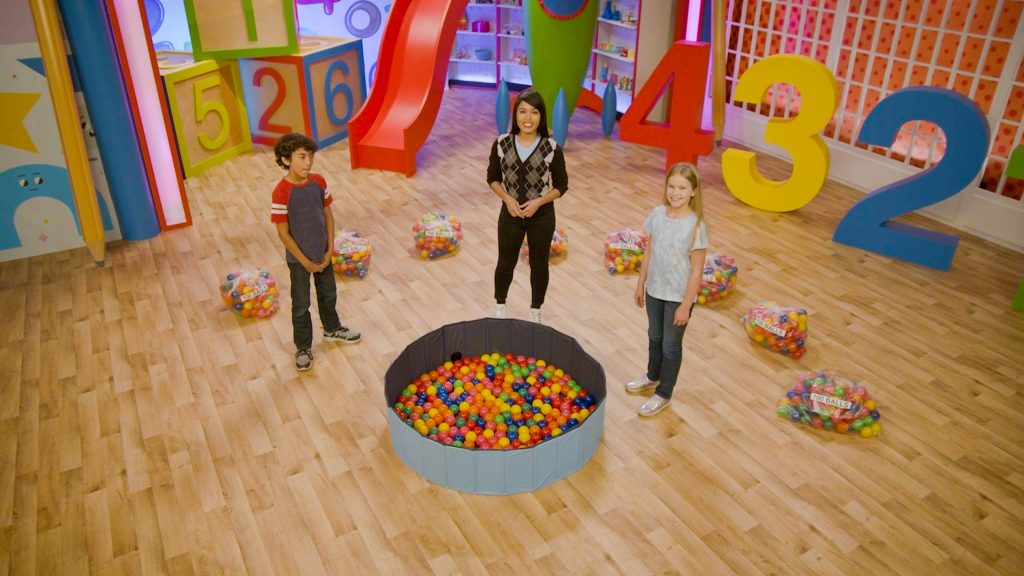WHAT IS SKIP COUNTING?

You can count more efficiently by skip counting. When you skip count, you skip some numbers while you count. You can skip count by 2s, 5s, 10s, and 100s, starting from any number.

To better understand skip counting…

WHAT IS SKIP COUNTING?. You can count more efficiently by skip counting. When you skip count, you skip some numbers while you count. You can skip count by 2s, 5s, 10s, and 100s, starting from any number. To better understand skip counting…

## LET’S BREAK IT DOWN!

### Review counting on a number line.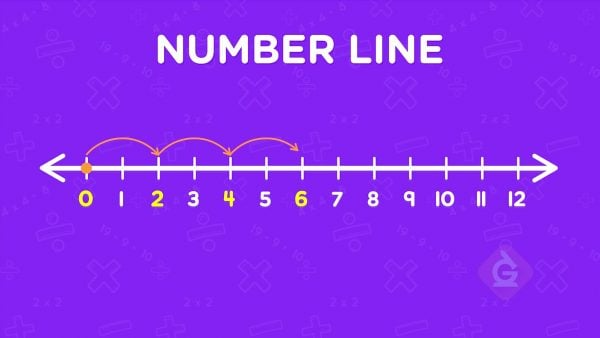A number line is a line that has arrows on both sides. It shows numbers in order. To count to 10 on a number line, you can start at 0, then jump to 1, then 2, then 3, 4, 5, 6, 7, 8, 9, 10. You could keep going forever because the number line never ends.

Review counting on a number line. A number line is a line that has arrows on both sides. It shows numbers in order. To count to 10 on a number line, you can start at 0, then jump to 1, then 2, then 3, 4, 5, 6, 7, 8, 9, 10. You could keep going forever because the number line never ends.

### Skip count basketball points by 2s.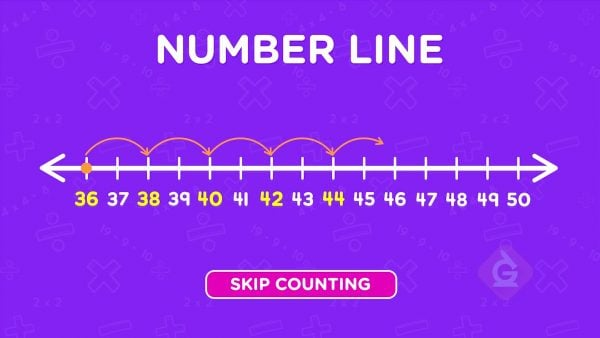In basketball, you can get 2 points for getting the ball in the hoop. Count the points as you score: 2, 4, 6, 8, 10, 12. Each time you count, you add 2. This is called skip counting because you skip some numbers while you count. When you skip count, you can start from any number. Start at 36 and skip count by 2s: 36, 38, 40, 42, 44.

Skip count basketball points by 2s. In basketball, you can get 2 points for getting the ball in the hoop. Count the points as you score: 2, 4, 6, 8, 10, 12. Each time you count, you add 2. This is called skip counting because you skip some numbers while you count. When you skip count, you can start from any number. Start at 36 and skip count by 2s: 36, 38, 40, 42, 44.

### Skip count bananas by 5s.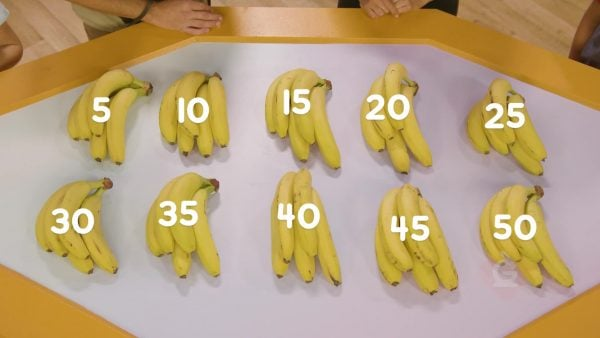You have some bunches of bananas. Each bunch has 5 bananas. You can skip count by 5s to count the bananas. 5, 10, 15, 20, 25, 30, 35, 40, 45, 50. Each number is 5 more than the number before it.

Skip count bananas by 5s. You have some bunches of bananas. Each bunch has 5 bananas. You can skip count by 5s to count the bananas. 5, 10, 15, 20, 25, 30, 35, 40, 45, 50. Each number is 5 more than the number before it.

### Skip count lollipops by 10s.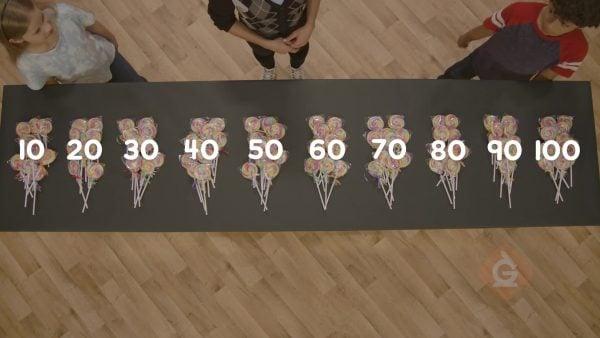You have a big bowl of lollipops. Put the lollipops in groups of 10. Skip count by 10s to count how many you have. 10, 20, 30, 40, 50, 60, 70, 80, 90, 100. Each number is 10 more than the number before it.

Skip count lollipops by 10s. You have a big bowl of lollipops. Put the lollipops in groups of 10. Skip count by 10s to count how many you have. 10, 20, 30, 40, 50, 60, 70, 80, 90, 100. Each number is 10 more than the number before it.

### Skip count balls by 100s.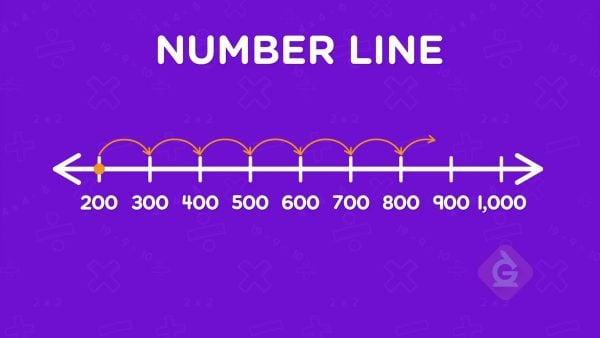There are 200 balls in a ball pit. You add several bags of 100 balls. Start at 200 and skip count by 100s. 300, 400, 500, 600, 700, 800, 900, 1,000.

Skip count balls by 100s. There are 200 balls in a ball pit. You add several bags of 100 balls. Start at 200 and skip count by 100s. 300, 400, 500, 600, 700, 800, 900, 1,000.

## SKIP COUNTING (2s, 5s, 10s & 100s) VOCABULARY

Number line
A line that has arrows on both sides and shows numbers in order. A number line never ends.
Skip counting
A more efficient way of counting where you skip some numbers each time you count. For example, if you skip count by 2s, you add 2 each time you count, so each time you skip one number on the number line.
Skip counting by 2s
To count 2 at a time. Each number said is 2 greater than the number before it.
Skip counting by 5s
To count 5 at a time. Each number said is 5 greater than the number before it.
Skip counting by 10s
To count 10 at a time. Each number said is 10 greater than the number before it.
Skip counting by 100s
To count 100 at a time. Each number said is 100 greater than the number before it.

## SKIP COUNTING (2s, 5s, 10s & 100s) DISCUSSION QUESTIONS

### What is skip counting?

Skip counting is a way of counting where you skip some numbers. For example, to skip count by 5, add 5 each time: 5, 10, 15, 20, …

### What do you notice about the numbers when you skip count by 2s?

I say every second number. Each number is 2 more than the last number.

### What do you notice about the numbers when you skip count by 5s?

When I skip count on a number chart, all of the numbers are in the center column or the end column. All of the numbers end in 5 or 0.

### What do you notice about the numbers when you skip count by 10s?

When I skip count on a number chart, all of the numbers are in the end column. All of the numbers end in 0. Each number is 10 more than the number before it. The tens digit goes up by 1 when I count until I get to a new hundred.

### What do you notice about the numbers when you skip count by 100s?

All of the numbers end in 00. The hundreds digit goes up by 1 when I count. Each number is 100 more than the number before it.
X

## Success

We’ve sent you an email with instructions how to reset your password.
Ok